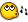## Recommended Posts

ok this is what i have but i want to make the loop working good, if i uncomment the loop and comment the ifs then it always shows the msgbox 10 times but it must only show it the times from 7 to \$second

get it?

```#include <Process.au3>
#include <File.au3>

FileDelete("output.txt")
\$countline = _FileCountLines("output.txt")

;~ for \$count = 10 to 20
;~ for \$lines = 8 to 18

;~ if \$countline = \$count Then
;~  \$second = \$lines
;~
;~ EndIf
;~ Next
;~ Next

if \$countline = 8 Then
\$second = 6
EndIf

if \$countline = 9 Then
\$second = 7
EndIf

if \$countline = 10 Then
\$second = 8
EndIf

if \$countline = 11 Then
\$second = 9
EndIf

if \$countline = 12 Then
\$second = 10
EndIf

if \$countline = 13 Then
\$second = 11
EndIf

if \$countline = 14 Then
\$second = 12
EndIf

if \$countline = 15 Then
\$second = 13
EndIf

if \$countline = 16 Then
\$second = 14
EndIf

if \$countline = 17 Then
\$second = 15
EndIf

if \$countline = 18 Then
\$second = 16
EndIf

if \$countline = 19 Then
\$second = 17
EndIf

if \$countline = 20 Then
\$second = 18
EndIf

for \$i = 7 to \$second
Next
FileDelete("output.txt")
EndFunc```
Edited by broodplank

##### Share on other sites

Try this:

```\$admins = ""
\$count = 0
\$objGroup = ObjGet("WinNT://" & @ComputerName & "/Administrators")
For \$objUser in \$objGroup.Members
\$count += 1
Next

[font="Fixedsys"][list][/list][/font]

##### Share on other sites

Or this is much faster:

```#include <Constants.au3>

\$foo = Run("net localgroup administrators", @SystemDir, @SW_HIDE, \$STDOUT_CHILD)
\$output = ""
While 1
If @error Then ExitLoop
Wend
\$aOutput = StringSplit(\$output,@crlf,1)

\$count = 0

\$valid = false
for \$i = 1 to \$aOutput
\$line = \$aOutput[\$i]
if stringinstr(\$line,"The command") then \$valid = false
if \$valid then
\$count += 1
EndIf
if stringinstr(\$line,"----------") then \$valid = true
Next

[font="Fixedsys"][list][/list][/font]

## Create an account

Register a new account

• ### Recently Browsing   0 members

×

• Wiki

• Back

• #### Beta

• Git
• FAQ
• Our Picks
×
• Create New...